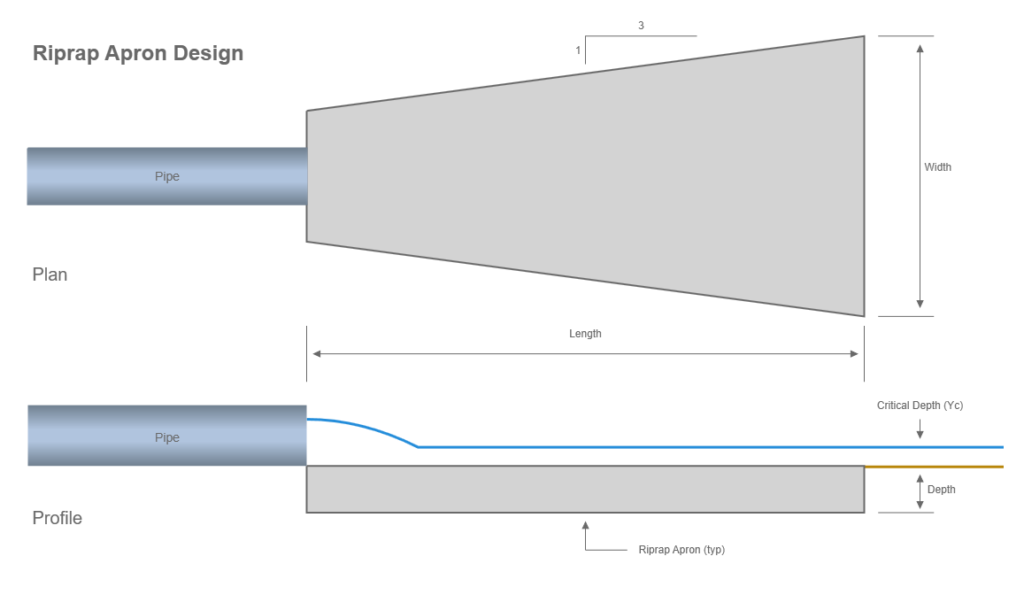# Riprap Apron Design

The most commonly used device for outlet protection is a riprap apron. An example schematic of an apron is shown below. The methods used here follow that of FHWA HEC-14, Third edition.They are constructed of riprap or grouted riprap at a zero grade for a distance that is related to the pipe’s Diameter or Rise. These aprons do not dissipate significant energy except through increased roughness for a short distance. However, they do serve to spread the flow, thus reducing velocity, helping to transition to the natural drainage way or to sheet flow where no natural drainage way exists.

The calculation procedure is fairly simple. The required riprap rock size, D50, is first computed. The apron dimensions are then pulled from a table.

The following equation is used to determine the Apron rock size, D50:Where:

D50 = required riprap rock size in ft (m)
Q = discharge in cfs (cms)
D = Pipe rise (diameter) in ft (m)
Tw = tailwater depth in ft (m)
g = acceleration due to gravity

Tailwater depth is limited to between 0.4D and 1.0D. For example, if Tw is less than 0.4D then Tw = 0.4D.

Once D50 is determined, it is converted to whole inches (mm). The Length and Depth dimensions are then taken from the following table:

ClassD50D50 (mm)Apron LengthApron Depth
151254D3.5D50
261504D3.3D50
3102505D2.4D50
4143506D2.2D50
5205007D2.0D50
6225508D2.0D50

The Apron Width is computed using a 3:1 flare as shown in the example schematic above. Once the final apron width is known, the downstream velocity is computed. This is computed by assuming critical depth at the apron end. If the Tailwater is greater then the Tailwater is used as the depth. Then velocity is computed as Q/A where A = Depth x Width.